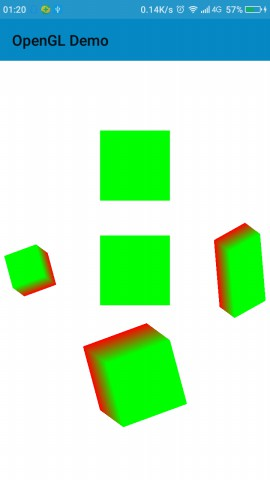# 什么是矩阵

x1y1z11=m1m2m3m4m5m6m7m8m9m10m11m12m13m14m15m16xyz1=m1x+m5y+m9z+m13m2x+m6y+m10z+m14m3x+m7y+m11z+m15m4x+m8y+m12z+m16(1)

# OpenGL中常用矩阵

1000010000100001()

100001000010δXδYδZ1()

10000cosβsinβ00sinβcosβ00001(X)

cosβ0sinβ00100sinβ0cosβ00001(Y)

cosβsinβ00sinβcosβ0000100001(Z)

Sx0000Sy0000Sz00001()

# 平移旋转缩放实例

public static void translateM(float[] m, int mOffset,float x, float y, float z) {
for (int i=0 ; i<4 ; i++) {
int mi = mOffset + i;
m[12 + mi] += m[mi] * x + m[4 + mi] * y + m[8 + mi] * z;
}
}

public class VaryTools {

private float[] mMatrixCamera=new float;    //相机矩阵
private float[] mMatrixProjection=new float;    //投影矩阵
private float[] mMatrixCurrent=     //原始矩阵
{1,0,0,0,
0,1,0,0,
0,0,1,0,
0,0,0,1};

private Stack<float[]> mStack;      //变换矩阵堆栈

public VaryTools(){
mStack=new Stack<>();
}

//保护现场
public void pushMatrix(){
mStack.push(Arrays.copyOf(mMatrixCurrent,16));
}

//恢复现场
public void popMatrix(){
mMatrixCurrent=mStack.pop();
}

public void clearStack(){
mStack.clear();
}

//平移变换
public void translate(float x,float y,float z){
Matrix.translateM(mMatrixCurrent,0,x,y,z);
}

//旋转变换
public void rotate(float angle,float x,float y,float z){
Matrix.rotateM(mMatrixCurrent,0,angle,x,y,z);
}

//缩放变换
public void scale(float x,float y,float z){
Matrix.scaleM(mMatrixCurrent,0,x,y,z);
}

//设置相机
public void setCamera(float ex,float ey,float ez,float cx,float cy,float cz,float ux,float uy,float uz){
Matrix.setLookAtM(mMatrixCamera,0,ex,ey,ez,cx,cy,cz,ux,uy,uz);
}

public void frustum(float left,float right,float bottom,float top,float near,float far){
Matrix.frustumM(mMatrixProjection,0,left,right,bottom,top,near,far);
}

public void ortho(float left,float right,float bottom,float top,float near,float far){
Matrix.orthoM(mMatrixProjection,0,left,right,bottom,top,near,far);
}

public float[] getFinalMatrix(){
float[] ans=new float;
Matrix.multiplyMM(ans,0,mMatrixCamera,0,mMatrixCurrent,0);
Matrix.multiplyMM(ans,0,mMatrixProjection,0,ans,0);
return ans;
}

}

GLES20.glClear(GLES20.GL_COLOR_BUFFER_BIT| GLES20.GL_DEPTH_BUFFER_BIT);
cube.setMatrix(tools.getFinalMatrix());
cube.drawSelf();

//y轴正方形平移
tools.pushMatrix();
tools.translate(0,3,0);
cube.setMatrix(tools.getFinalMatrix());
cube.drawSelf();
tools.popMatrix();

//y轴负方向平移，然后按xyz->(0,0,0)到(1,1,1)旋转30度
tools.pushMatrix();
tools.translate(0,-3,0);
tools.rotate(30f,1,1,1);
cube.setMatrix(tools.getFinalMatrix());
cube.drawSelf();
tools.popMatrix();

//x轴负方向平移，然后按xyz->(0,0,0)到(1,-1,1)旋转120度，在放大到0.5倍
tools.pushMatrix();
tools.translate(-3,0,0);
tools.scale(0.5f,0.5f,0.5f);

//在以上变换的基础上再进行变换
tools.pushMatrix();
tools.translate(12,0,0);
tools.scale(1.0f,2.0f,1.0f);
tools.rotate(30f,1,2,1);
cube.setMatrix(tools.getFinalMatrix());
cube.drawSelf();
tools.popMatrix();

//接着被中断的地方执行
tools.rotate(30f,-1,-1,1);
cube.setMatrix(tools.getFinalMatrix());
cube.drawSelf();
tools.popMatrix();# 源码07-158954
06-024839
02-20118
08-08171
07-136262
01-08166
04-122086
07-08797
07-10
11-13313
05-301998
07-254049
05-12
10-162293
09-068955
08-214106